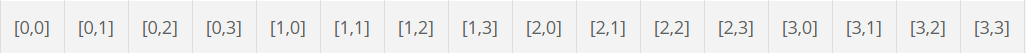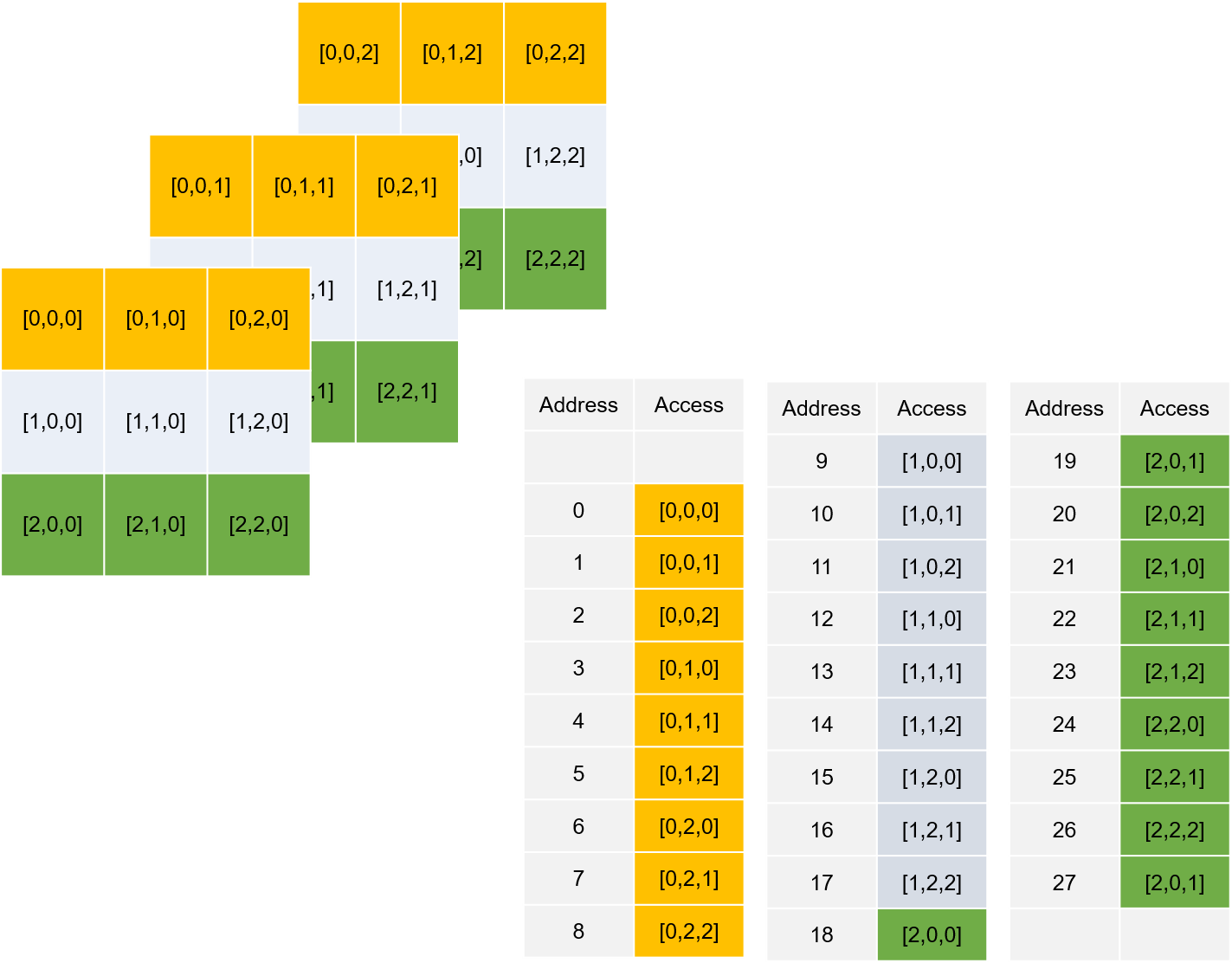# Image Processing 101: What is an Image?

Image processing and computer vision are hot trends in computer science that continue to show strong signs of momentum into the future due to their wide application and optimistic development prospects. In the first post of this blog series, we introduce the fundamentals of image processing by looking at what are images and how images are stored.

Digital images can be displayed and processed on a computer and can be divided into two broad categories based on their characteristics – bitmaps and vector images.

Bitmaps are based on pixel patterns that are usually represented by a digital array. BMP, PNG, JPG, and GIF are bitmaps.Vector images an infinitely scalable and do not have any pixels since they use mathematical formulas to draw lines and curves. A bitmap image of size M × N is composed of finite elements of M rows and N columns. Each element has a specific position and amplitude, representing the information at the position, such as grayscale and color. These elements are called image elements or pixels.

## Color Space

Depending on the information represented by each pixel, images can be divided into binary images, grayscale images, RGB images, and index images, etc.

1. Binary Image

In a binary image, the pixel value is represented by a 0 or 1. Generally, 0 is for black and 1 is for white.

1. Grayscale Image

The grayscale image adds a color depth between black and white in the binary image to form a grayscale image. Such images are usually displayed as grayscales from the darkest black to the brightest white, and each color depth is called a grayscale, usually denoted by L. In grayscale images, pixels can take integer values between 0 and L-1.

1. RGB Image

In RGB or color, images, the information for each pixel requires a tuple of numbers to represent. So we need a three-dimensional matrix to represent an image. Almost all colors in nature can be composed of three colors: red (R), green (G), and blue (B). So each pixel can be represented by a red/green/blue tuple in an RGB image.

1. Indexed Image

An indexed image consists of a colormap matrix, which uses direct mapping of pixel values in an array to colormap values. The color of each pixel in an image is determined by using the corresponding value. We discuss this in more detail below.

## How an Image is Stored in Memory

The x86 hardware does not have an addressing mode that accesses elements of multi-dimensional arrays. When loading an image into memory space, the multi-dimensional object is converted into a one-dimensional array. Row major ordering or column major ordering are commonly used.

### Row Major Ordering

C/C++ and Python employ row-major ordering. It starts with the first row and then concatenates the second row to its end, then the third row, etc. This means that in a row-major layout, the last index is the fastest changing. In the case of matrices, the last index is columns.

For grayscale images, we can use a matrix to represent the level of gray at each pixel. Take a 4*4 image for example.

Pixels by row and column:

 [0,0] [0,1] [0,2] [0,3] [1,0] [1,1] [1,2] [1,3] [2,0] [2,1] [2,2] [2,3] [3,0] [3,1] [3,2] [3,3]

Memory:For color images, we need multi-dimensional arrays to store the image information.### Column-Major Ordering

In the row-major layout of multi-dimensional arrays, the first index is the fastest changing. Below is an example.

Image A with 6 pixels:

 A A A A A A

With the row-major order, the consecutive memory address will be allocated like this:

 Address Access 0 A 1 A 2 A 3 A 4 A 5 A

With the column-major order, the consecutive memory address will be allocated like this:

 Address Access 0 A 1 A 2 A 3 A 4 A 5 A

## How an Image is Stored in Files

### True Color (24-bit)

24-bit images commonly use 8 bits of each of R, G, and B. For each of the three primary colors, like grayscale, L levels can be used to indicate how much of this color component is present. For example, for a red color with 256 levels, 0 means no red color and 255 means 100% red. Similarly, green and blue can be divided into 256 levels. Each primary color can be represented by an 8-bit binary data, so the total of 3 primary colors requires 24 bits.

The uncompressed raw BMP file is an RGB image stored using the RGB standard.

### Indexed Color

For a color image with a height and width of 200 pixels and 16 colors, each pixel is represented by three components of RGB. Thus each pixel is represented by 3 bytes and the entire image is 200 × 200 × 3 = 120KB. Since there are only 16 colors in the color image, you can save the RGB values of these 16 colors with a color table (a 16 × 3 two-dimensional array). We discuss this in further detail below. Every element in the array represents a color, indexed by its position within the array. The image pixels do not contain the full specification of its color, but only its index in the table. For example, if the third element in the color table is 0xAA1111, then all pixels with a color of 0xAA1111 can be represented by “2” (the color table index subscript starts at 0). This way, each pixel requires only 4 bits (0.5 bytes), so the entire image can be stored at 200 × 200 × 0. 5 = 20 KB. The color table referred to above is the palette, which is also often called Look Up Table (LUT).

GIF is the most representative image file format that supports indexed color modes.There are many other image formats and storage schemes. We will not get into the details of them for now. Subscribe to our newsletter to learn more in our Image Processing Series.  Next, we will discuss color models.